# NCERT Solutions for Class 6 Maths Chapter 9 Data Handling

## NCERT Solutions for Class 6 Maths Chapter 9 Data Handling

Today in our article you will be told that when your ncert solutions for class 6 maths Chapter 9 Data Handling will be released and you will be provided complete information about it. In our article, you will also be given complete information about the model paper. Also, you will be provided complete information about the model paper download process in our article. So please read it carefully till the end and stay connected with our website till the end.

ncert solutions for class 6 maths Chapter 9 Data Handling

## Exercise 9.1

1. In a Mathematics test, the following marks were obtained by 40 students. Arrange these marks in a table using tally marks.

 8 1 3 7 6 5 5 4 4 2 4 9 5 3 7 1 6 5 2 7 7 3 8 4 2 8 9 5 8 6 7 4 5 6 9 6 4 4 6 6

(a) Find how many students obtained marks equal to or more than 7.

(b) How many students obtained marks below 4?

Solutions:

 Marks Tally Marks Number of Students 1 || 2 2 ||| 3 3 ||| 3 4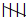|| 7 5| 6 6|| 7 75 8 |||| 4 9 ||| 3

(a) The students who got marks equal to or more than 7 are the students who got marks as either of 7, 8, and 9. Therefore number of these students

= 5 + 4 + 3

= 12

(b) The students who got marks below 4 are the students who got marks as either of 1, 2, and 3. Therefore number of these students are

= 2 + 3 + 3

= 8

2. Following is the choice of sweets of 30 students of Class VI.

(a) Arrange the names of sweets in a table using tally marks.

(b) Which sweet is preferred by most of the students?

Solutions:

By observing the choice of sweets of 30 students. We may construct the table as shown below:

 Sweets Tally Marks Number of Students Ladoo| 11 Barfi ||| 3 Jalebi|| 7 Rasgulla|||| 9 30

(b) The highest number of students preferred Ladoos. Hence, Ladoo is the most preferred sweet among students.

3. Catherine threw a dice 40 times and noted the number appearing each time as shown below :

 1 3 5 6 6 3 5 4 1 6 2 5 3 4 6 1 5 5 6 1 1 2 2 3 5 2 4 5 5 6 5 1 6 2 3 5 2 4 1 5

Make a table and enter the data using tally marks. Find the number that appeared.

(a) The minimum number of times

(b) The maximum number of times

(c) Find those numbers that appear an equal number of times.

Solutions:

 Numbers Tally Marks Number of times 1|| 7 2| 6 35 4 |||| 4 5| 11 6|| 7

(a) The number that occurred for minimum number of times is 4

(b) The number that occurred for maximum number of times is 5

(c) 1 and 6 are the numbers that appear an equal number of times.

4. Following pictograph shows the number of tractors in five villages.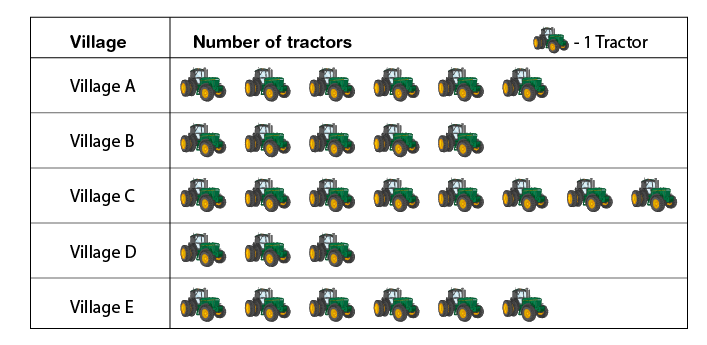Observe the pictograph and answer the following questions.

(i) Which village has the minimum number of tractors?

(ii) Which village has the maximum number of tractors?

(iii) How many more tractors village C has as compared to village B?

(iv) What is the total number of tractors in all five villages?

Solutions:

(i) Village D has the minimum number of tractors.

(ii) Village C has the maximum number of tractors.

(iii) Village B has 5 tractors

Village C has 8 tractors

= 8 – 5

= 3 tractors

Village C has 3 more tractors as compared to village B

(iv) Total number of tractors in all the villages = 6 + 5 + 8 + 3 + 6 = 28 tractors

5. The number of girl students in each class of a co-educational middle school is depicted by the pictograph: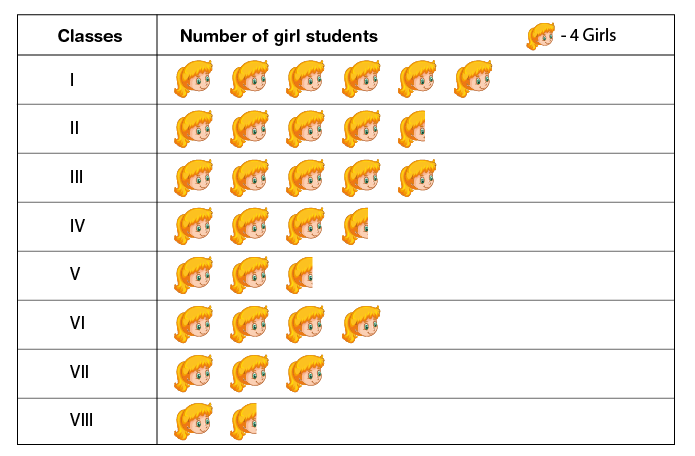Observe this pictograph and answer the following questions:

(a) Which class has the minimum number of girl students?

(b) Is the number of girls in Class VI less than the number of girls in Class V?

(c) How many girls are there in Class VII?

Solutions:

By observing the above table, there are 24, 18, 20, 14, 10, 16, 12, and 6 girls respectively from class I to VIII

(a) Class VIII has only 6 girls. Therefore, the minimum number of girl students are in Class VIII

(b) No. Class V has 10 girl students

Class VI has 16 girl students

Hence, the number of girls in Class VI are more than the number of girls in Class V

(c) The number of girls in Class VII are 12

6. The sale of electric bulbs on different days of a week is shown below: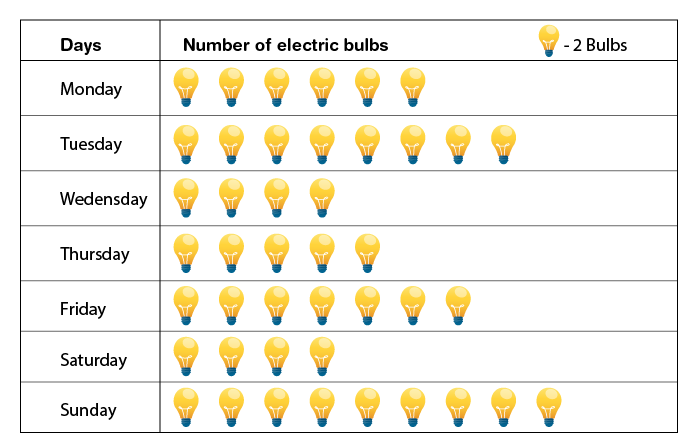Observe the pictograph and answer the following questions:

(a) How many bulbs were sold on Friday?

(b) On which day were the maximum number of bulbs sold?

(c) On which of the days same number of bulbs were sold?

(d) On which of the days minimum number of bulbs were sold?

(e) If one big carton can hold 9 bulbs. How many cartons were needed in the given week?

Solutions:

(a) Number of bulbs sold on Friday are 14 bulbs.

(b) On Sunday highest number of bulbs i.e 18 are sold. Thus maximum number of bulbs were sold on Sunday.

(c) On Wednesday and Saturday 8 bulbs are sold. Hence equal number of bulbs were sold on Wednesday and Saturday.

(d) Minimum number of bulbs were sold on Wednesday and Saturday i.e 8 bulbs.

(e) Total number of bulbs sold in a week = 12 + 16 + 8 + 10 + 14 + 8 + 18 = 86

7. In a village six fruit merchants sold the following number of fruit baskets in a particular season: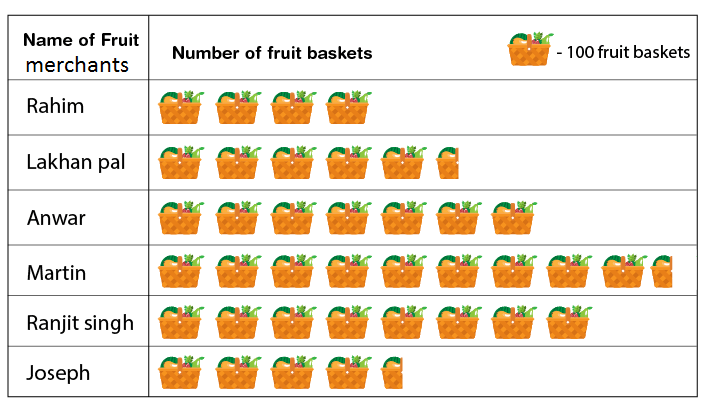Observe this pictograph and answer the following questions:

(a) Which merchant sold the maximum number of baskets?

(b) How many fruit baskets were sold by Anwar?

(c) The merchants who have sold 600 or more number of baskets are planning to buy a godown for the next season. Can you name them?

Solutions:

From the pictograph, the number of fruit baskets sold by Rahim, Lakhanpal, Anwar, Martin, Ranjit Singh and Joseph are 400, 550, 700, 950, 800 and 450 respectively

(a) Martin sold the maximum number of fruit baskets i.e 950

(b) Anwar sold 700 fruit baskets

(c) Anwar, Martin, and Ranjit Singh are the merchants who sold more than 600 fruit baskets. Hence, these are the merchants who are planning to buy a godown for the next season.

## Exercise 9.2

1. the Total number of animals in five villages are as follows:

Village A: 80

Village B: 120

Village C: 90

Village D: 40

Village E: 60

Prepare a pictograph of these animals using one symbol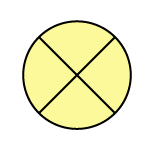to represent 10 animals and answer the following questions:

(a) How many symbols represent animals of village E?

(b) Which village has the maximum number of animals?

(c) Which village has more animals: village A or village C?

Solutions:

We can draw the pictograph for the given data as shown below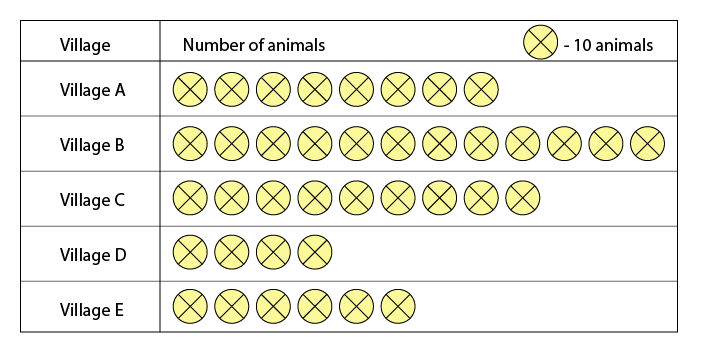(a) There are 60 animals in village E. So 6 symbols represent animals of village E

(b) Village B has 120 animals which is the maximum number among these villages.

(c) Village A has 80 animals and village C has 90 animals. Clearly, village C has more animals than village A

2. Total number of students of a school in different years is shown in the following table

 Years Number of Students 1996 400 1998 535 2000 472 2002 600 2004 623

A. Prepare a pictograph of students using one symbolto represent 100 students and answer the following questions:

(a) How many symbols represent the total number of students in the year 2002?

(b) How many symbols represent the total number of students for the year 1998?

B. Prepare another pictograph of students using any other symbol each representing 50 students. Which pictograph do you find more informative?

Solutions: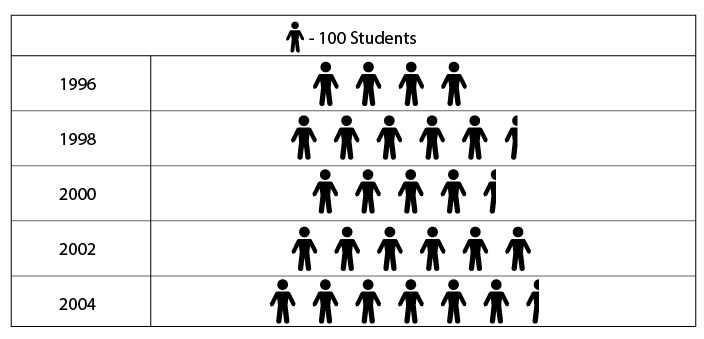A

(a) Total number of students in the year 2002 represents 6 symbols

(b) Total number of students in the year 1998 represents 5 complete and 1 incomplete symbol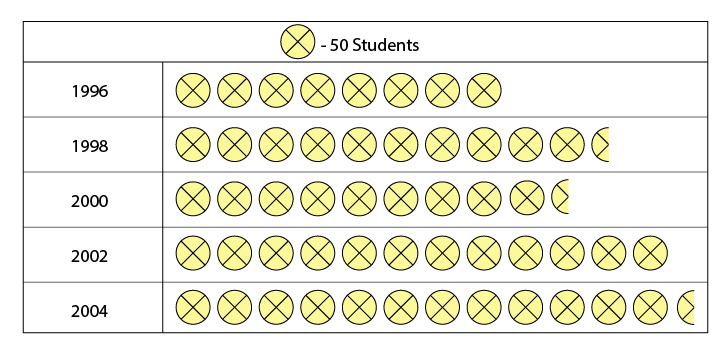## Exercise 9.3

1. The bar graph given alongside shows the amount of wheat purchased by the government during the year 1998-2002.

Read the bar graph and write down your observations. In which year was

(a) the wheat production maximum?

(b) the wheat production minimum?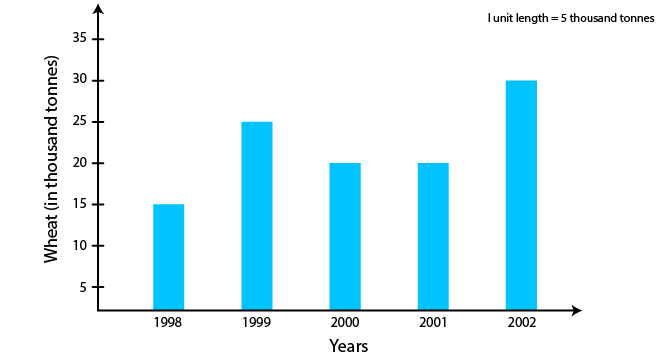Solutions:

(a) The wheat production was maximum in the year 2002

(b) The wheat production was minimum in the year 1998

2. Observe this bar graph which is showing the sale of shirts in a ready-made shop from Monday to Saturday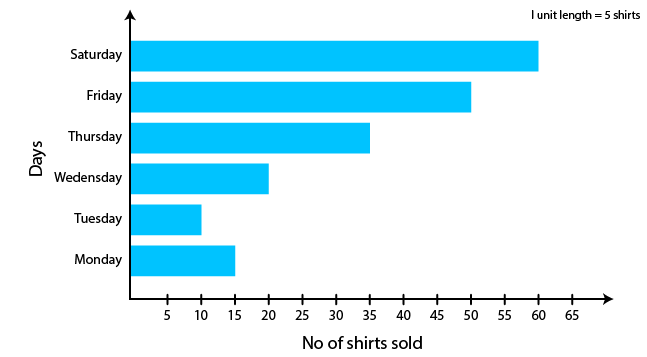(a) What information does the above bar graph give?

(b) What is the scale chosen on the horizontal line representing the number of shirts?

(c) On which day were the maximum number of shirts sold? How many shirts were sold on that day?

(d) On which day were the minimum number of shirts sold?

(e) How many shirts were sold on Thursday?

Solutions:

(a) The above bar graph shows the number of shirts sold from Monday to Saturday

(b) 1 unit length = 5 shirts is the scale on the horizontal line representing the number of shirts.

(c) On Saturday maximum number of shirts sold i.e 60 shirts were sold.

(d) On Tuesday minimum number of shirts sold i.e 10 shirts were sold.

(e) 35 shirts were sold on Thursday

3. Observe this bar graph which shows the marks obtained by Aziz in half-yearly examination in different subjects. Answer the given questions.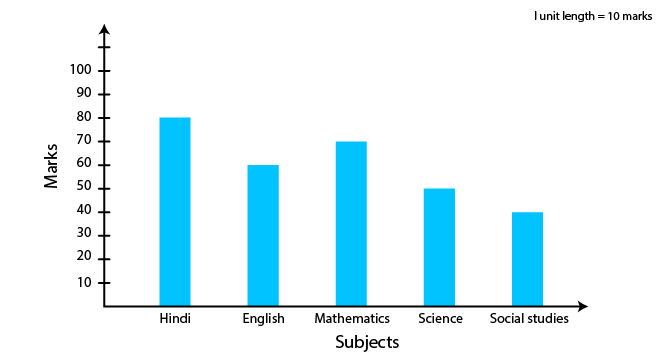(a) What information does the bar graph give?

(b) Name the subject in which Aziz scored maximum marks.

(c) Name the subject in which he has scored minimum marks.

(d) State the name of the subjects and marks obtained in each of them.

Solutions:

(a) The bar graph shows the marks scored by Aziz in different subjects.

(b) Aziz scored maximum marks in Hindi i.e 80 marks

(c) Aziz scored minimum marks in Social Studies i.e 40 marks

(d) Hindi – 80 marks English – 60 marks Mathematics – 70 marks

Science – 50 marks Social Studies – 40 marks

## Exercise 9.4

1. A survey of 120 school students was done to find which activity they prefer to do in their free time.

 Preferred activity Number of Students Playing 45 Reading storybooks 30 Watching T.V 20 Listening to music 10 Painting 15

Draw a bar graph to illustrate the above data taking a scale of 1 unit length = 5 students. Which activity is preferred by most of the students other than playing?

Solutions:

By taking a scale of 1 unit length = 5 students, a bar graph is shown below considering the above data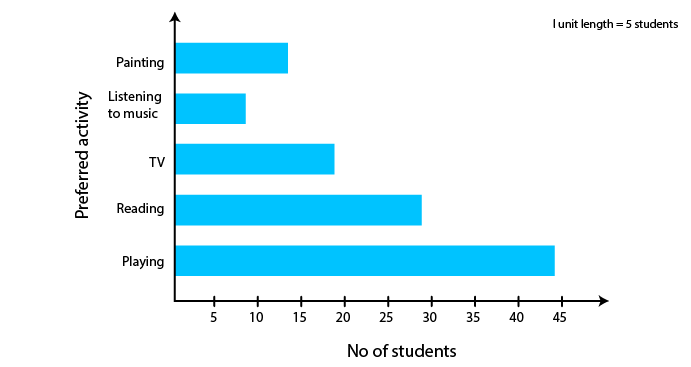Reading storybooks is the activity preferred by most of the students other than playing

2. The number of Mathematics books sold by a shopkeeper on six consecutive days is shown below:

 Days Sunday Monday Tuesday Wednesday Thursday Friday Number of books sold 65 40 30 50 20 70

Draw a bar graph to represent the above information choosing the scale of your choice.

Solutions:

By taking a scale of 1 unit length = 10 books we may draw a bar graph of the above data as follows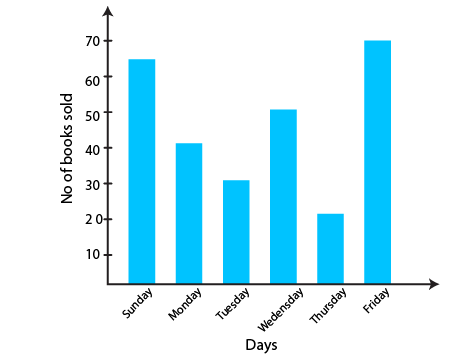3. Following table shows the number of bicycles manufactured in a factory during the years 1998 to 2002. Illustrate this data using a bar graph. Choose a scale of your choice

 Year Number of bicycles manufactured 1998 800 1999 600 2000 900 2001 1100 2002 1200

(a) In which year was the maximum number of bicycles manufactured?

(b) In which year was the minimum number of bicycles manufactured?

Solutions:

By taking a scale of 1 unit length = 100 bicycles we may draw a bar graph of the above data as follows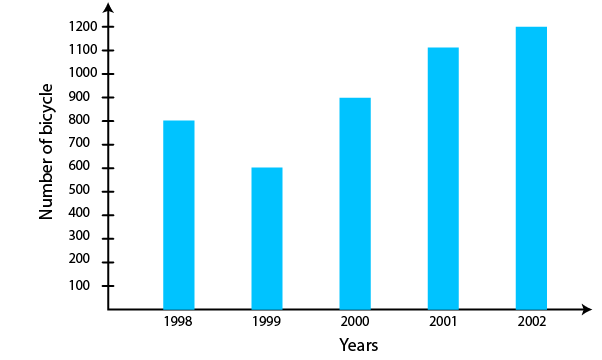(a) In the year 2002, the maximum number of bicycles were manufactured i.e 1200 bicycles

(b) In the year 1999, the minimum number of bicycles were manufactured i.e 600 bicycles

4. Number of persons in various age groups in a town is given in the following table.

 Age group(in years) 1-14 15-29 30-44 45-59 60-74 75 and above Number of persons 2 lakhs 1 lakh 60 thousand 1 lakh 20 thousand 1 lakh 20 thousand 80 thousand 40 thousand

Draw a bar graph to represent the above information and answer the following questions. (take 1 unit length = 20 thousands)

(a) Which two age groups have same population?

(b) All persons in the age group of 60 and above are called senior citizens. How many senior citizens are there in the town?

Solutions:

By taking a scale of 1 unit length = 20 thousand we may draw a bar graph of the above data as follows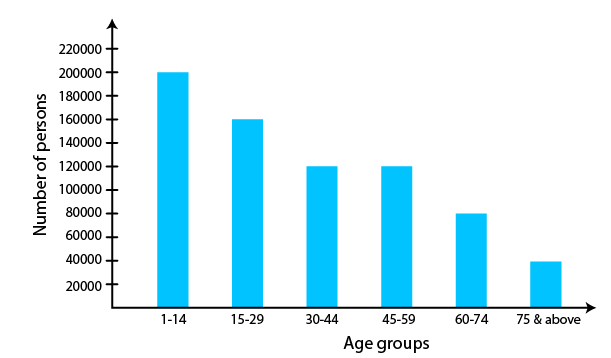(a) The two age groups which have same population are 30-44 and 45-59

(b) Senior citizens are persons between the age group either from 60-74 or from 75 above. Therefore

Number of senior citizens in the town = 80000 + 40000

= 1 lakh 20 thousand

Hence, the number of senior citizens in the town are 1 lakh 20 thousand

I hope you like the information and notes given by us. This will help you a lot in your upcoming exams. Here we are giving ncert solutions to all of you students, through which all of you can score very well in your exams. In this post, we are giving you ncert solutions for class 6 maths. hope you benefit from it. You can download the pdf file of this ncert solutions.

 Whatsapp Group Join
 Telegram channel Join